# Solving & Graphing Linear Equations Chapter Exam

Exam Instructions:

Choose your answers to the questions and click 'Next' to see the next set of questions. You can skip questions if you would like and come back to them later with the yellow "Go To First Skipped Question" button. When you have completed the practice exam, a green submit button will appear. Click it to see your results. Good luck!

### Page 3

#### Question 11 11. If the graph below has the equation Ax+By=C, which statement MUST be true?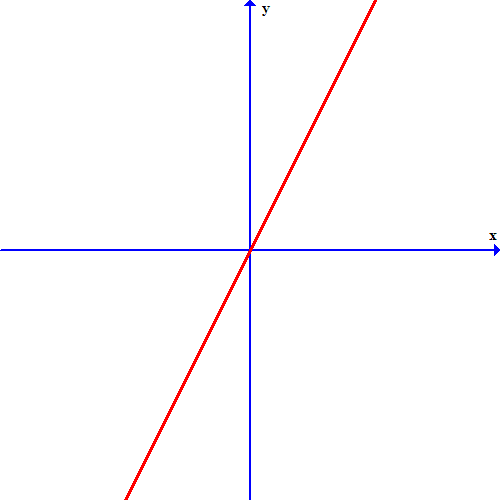### Page 4

#### Question 16 16. Which line is the transversal?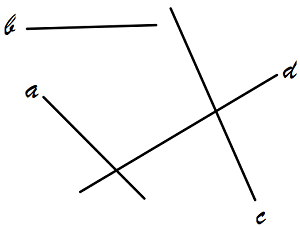#### Question 18 18. What is the equation of the graph below?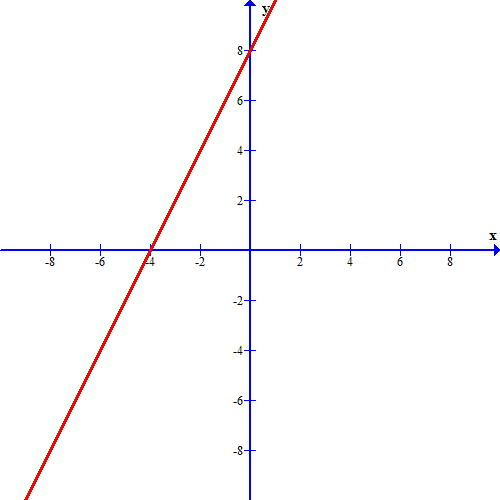### Page 6

#### Question 28 28. Which one of the following equations could work for the following graph?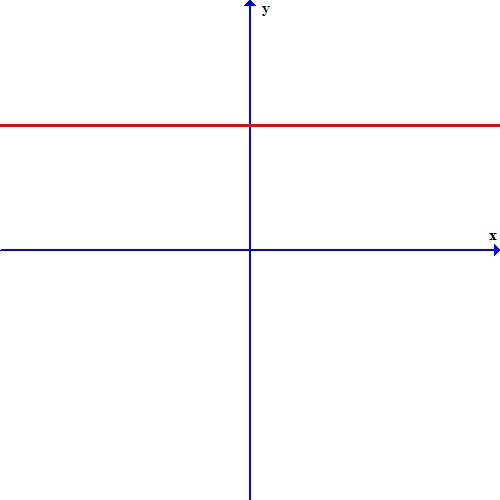#### Question 29 29. Which linear equation is shown below?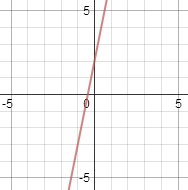#### Question 30 30. What is the slope-intercept form equation of the graph below?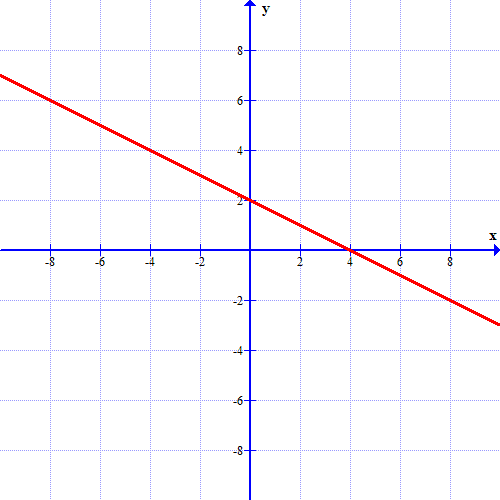#### Solving & Graphing Linear Equations Chapter Exam Instructions

Choose your answers to the questions and click 'Next' to see the next set of questions. You can skip questions if you would like and come back to them later with the yellow "Go To First Skipped Question" button. When you have completed the practice exam, a green submit button will appear. Click it to see your results. Good luck!

Support